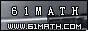Today is . WELCOME: huohai3983/44663  Home | Reg Login | All user | setHomePage | BookmarkHome | Math Test Online | Addition | Subtraction | Multiplication | Division | Time | Worksheet(JPG/PDF) | CAI | Flash Games |Recommendation articleArticle searchHome--maths--Fourth Grade--Subtracting Money

Subtracting Money

Subtracting Money

Amounts of money may be written in several different ways. Cents may be written with the ?sign and dollars can be written with the dollar sign (\$). Subtracting money that is expressed in these forms just involves subtracting the amounts and placing the proper sign on the answer.

Often money is written as a decimal with dollars to the left of the decimal point and cents to the right of the decimal point. Twenty-three dollars and eighty-seven cents is written \$23.87.

Decimal money amounts are subtracted the same way that decimals are subtracted. Remember to put the \$ sign before the answer.

Subtracting Decimals is just like subtracting other numbers.

Always line up the decimal points when subtracting decimals.

Remember to put the decimal point in the proper place in your answer.

#### What is the Difference between the two Amounts?

 -     =   \$

2007-01-18 20:18:53
Viewed 2411 times CloseGreatmathsites

### Translate

 Thank you to online math channels' Mathematics Department for hosting these lessons. Copyright © 2006 - 2008 GZtrain Inc. All Rights Reserved